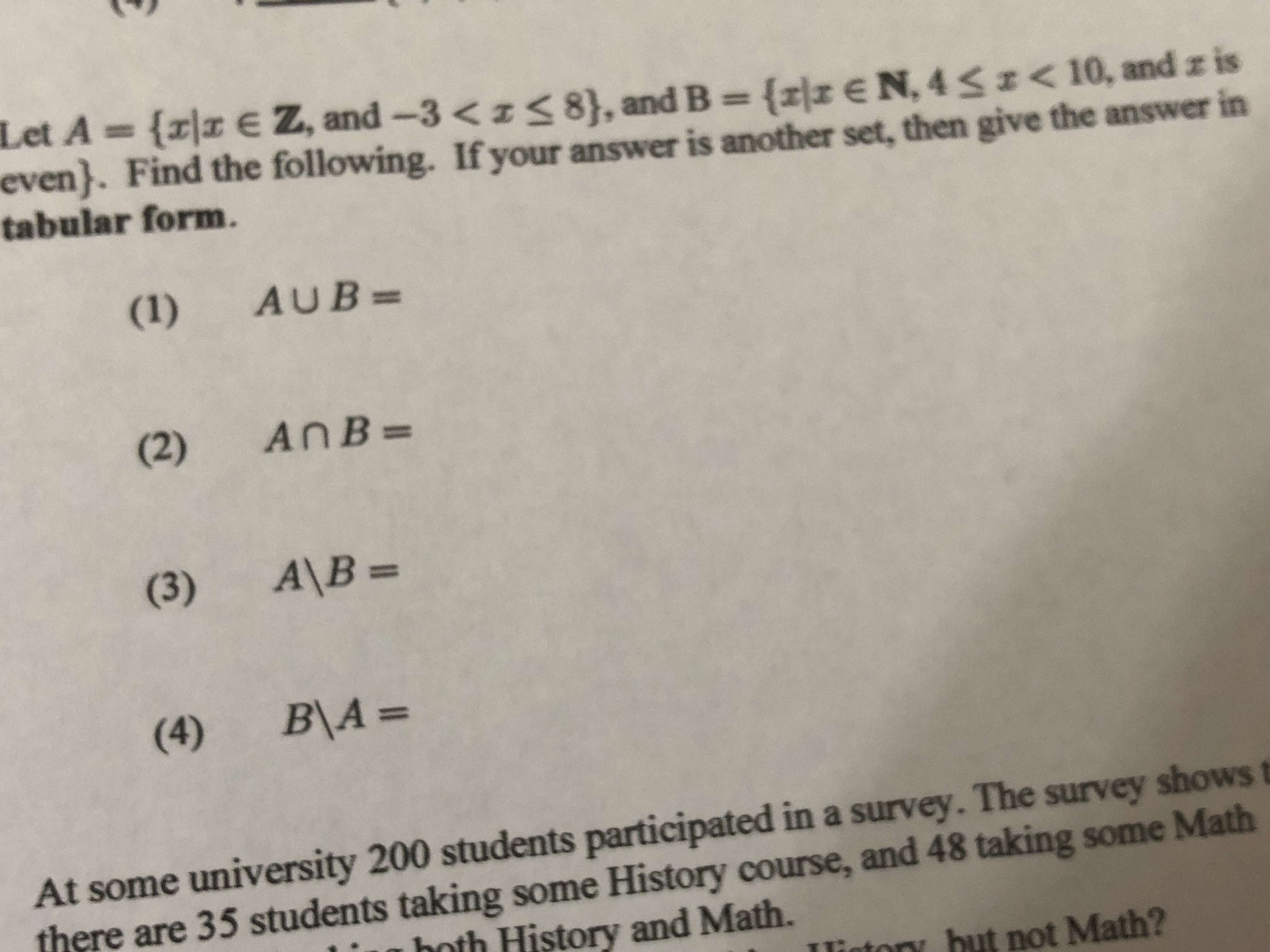EN.4Szc 10, and z iseven). Find the following. If your answer is another set, then give the answer in(1)AUB=(2)AnB(4)BM =At some university 200 students participated in a survey. The survey shows tthere are 35 students taking some History course, and 48 taking some Mathhoth History and MathIlintory hut not Math?

Questionhelp_outlineImage TranscriptioncloseEN.4Szc 10, and z is even). Find the following. If your answer is another set, then give the answer in (1) AUB= (2) AnB (4) BM = At some university 200 students participated in a survey. The survey shows t there are 35 students taking some History course, and 48 taking some Math hoth History and Math Ilintory hut not Math? fullscreen
Step 1

Consider the set builder form

Step 2

Convert the set A and B into tabular form as follows.

Step 3

Part (1):

The union of the sets...

Want to see the full answer?

See Solution

Want to see this answer and more?

Our solutions are written by experts, many with advanced degrees, and available 24/7

See Solution
Tagged in

Algebra